# Load Pull for Power Devices

Click here to go to our page on efficiency of microwave devices

Here's a page on the relative merits of in-phase and quadrature combined amplifiers with respect to immunity to load-pull.

Steve Cripps' method for load-line analysis of power amplifiers is often used in absence of, or to corroborate load pull data.

Load pull involves varying the load impedance presented to a device under test and monitoring a single or set of performance parameters.  When used in conjunction with a signal source and signal analyzer (spectrum analyzer, power meter, vector receiver…), load pull can be used to measure parameters such as output power, gain, and efficiency as a function of load impedance presented to the DUT.

For a nice summary of load pull, you may want to watch this video from our friends over at Keysight.  It covers load pull basics, and shows you how to set up and run load pull simulations on their ADS software.

In its simplest form, there are only four steps to load pull:

1. Vary impedance presented to DUT
2. Measure parameter (power, gain…)
3. Determine best matching impedance
4. Design matching network (using ADS for example)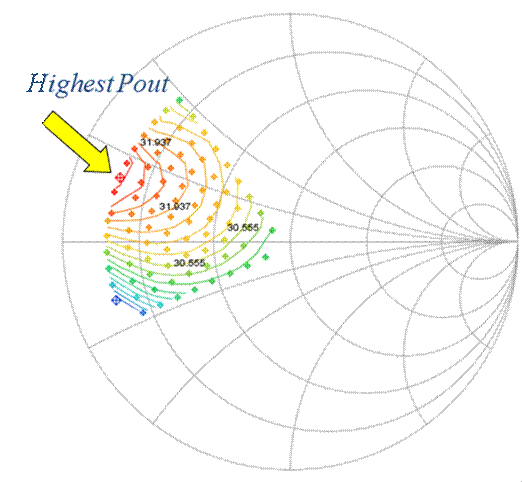Smith chart display of load pull

The impedance presented to the DUT can be stated in various formats: Impedance ZL (consisting of R+jX), VSWR (a real number) and its associated phase angle (thanks for the correction, Joe!), and ΓL (as a complex number in magnitude and phase). Considering our DUT as a two-port device shown in Figure 1, ΓL is nothing more than a2/b2, or the ratio between the reflected- and forward-traveling waves. A generalized form of the formula can be written as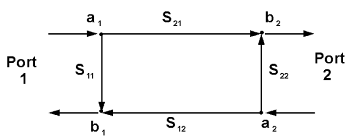The first and most common method uses impedance tuners to physically impede the flow of energy through the tuner and reflect part of the signal back to the DUT.  Because the a-wave  is a partial reflection of the b-wave, a2 will always be less than b2, limiting our reflection coefficient Gamma to a value less than 1.  Increased losses between the tuner and DUT from cables, probes, fixtures… will further limit the amount of energy reflected as a2 and reduce gamma even more.  Typical values range between ΓL=0.8 and ΓL=0.92 at the device reference plane.

A second method separates the forward traveling wave b from the reverse traveling wave a. Instead of reflecting a portion of b back as a, actually a new signal is created using a signal source with variable magnitude and phase control.  This approach is commonly referred to as open-loop active load pull.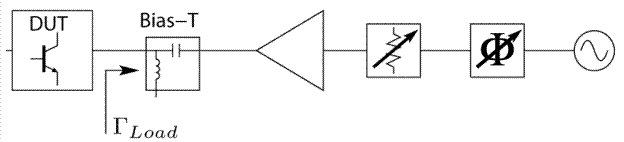In their most basic forms, traditional load pull systems comprise of a signal source used to generate a test signal, an input tuner to vary the source impedance seen by the DUT, an output tuner to vary the load impedance seen by the DUT, and a power meter to measure the resulting power.  This system relies heavily on a scalar instrument, the power meter, to measure output power which is then de-embedded to the DUT reference plane.

The advantage of a traditional load pull system lies in the flexibility of the components used: a low-budget power meter only system with a single tuner can be easily and inexpensively assembled, or a more complex system using advanced instrumentation can be selected.  Additionally, harmonic power levels and intermodulation products can be measured by adding a spectrum analyzer to the output of the setup.  Lastly, modulated signals are easily accommodated by using a vector-signal generator and vector-spectrum analyzer.

The disadvantage of a traditional load pull system lies in the scalar nature of the instruments used.  Vector-based parameters such as AM/PM cannot be measured without adding a dedicated network analyzer into the setup.  Additionally, the accuracy of the measurements relies completely on the accurate and reliable de-embedding of the output power through the passive components that lie between the power meter and load tuner, as well as the accurate and reliable de-embedding through the output tuner to reach the DUT reference plane.  Lastly, the input power made available to the DUT is known, but the actual delivered power is not known since the mismatch between the source tuner and DUT is not quantified (the input impedance of the DUT is not known, and changes as a function of load impedance and input power).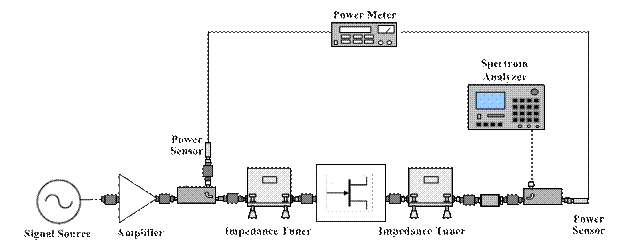Vector-Receiver load pull, also referred to as Real-Time load pull, replaces the scalar power meter and spectrum analyzer with a vector receiver.  Instead of measuring powers and de-embedding to the DUT reference plane, this methodology uses low-loss couplers between the tuners and DUT to sample the a1, a2, b1 and b2 waves at the DUT reference plane.  Useful parameters such as delivered input power, output power, power gain and PAE can be calculated using the formulas below.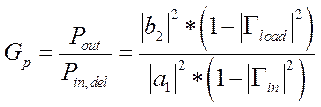Advantages of the vector-receiver load pull method include the inherent measurement of vector parameters including AM/PM.  Additionally, by using the a- and b-waves at the DUT reference plane, it is possible to measure the large signal input impedance of the DUT (which changes constantly as a function of load impedance presented to the DUT and input power) and therefore calculate the actual delivered power to the input of the device, not only the available power.  Measurement accuracies are enhanced as the a- and b-waves are sampled at the DUT reference plane; no de-embedding is required and no reliance is placed on the repeatability or accuracy of the impedance tuners.  Harmonic powers and intermodulation parameters are available as the a- and b-waves are measured at each frequency of interest.

The disadvantage of the vector-receiver load pull system lies in its inability to measure modulated signals.  Typical vector-receivers use narrow-band ADCs and are unable to decode wideband signals.  Additionally, the added losses of the couplers placed between the tuners and DUT may reduce the achievable VSWR to the DUT (that is why it is important to use low-loss couplers).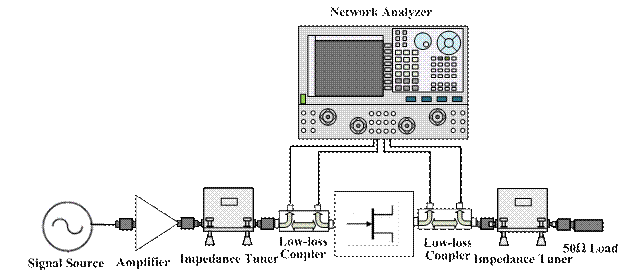Open-loop active load pull operates on the same principle as vector-receiver load pull, in that the a- and b-waves are measured and used to calculate powers, gain, efficiency…  However, instead of using mechanical impedance tuner(s) to vary the load impedance presented to the DUT, active tuning chains consisting of a signal source with variable magnitude and phase control (as described above) are used.  To present a specific impedance to the DUT, the b2-wave coming from the device is measured, and the required a2-wave is calculated using the formula: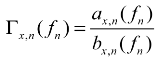An iterative process which can last tenths of seconds to several seconds will normally lead to a convergence at the requested impedance.

It is a simple feat to expand an open-loop active load pull system’s capabilities to harmonic load pull.  A multiplexer, combiner, coupler or other passive component can be used to merge active tuning chains at multiple frequencies(harmonic frequencies or other) in order to present controlled impedances at said frequencies to the DUT.

Advantages of open-loop active load pull include the potential of Gamma>1 (really large VSWR).  Gamma is directly related to the magnitude of the a2 wave, and the a2 wave can be increased by using a large amplifier in the active tuning chain.  Another advantage is the ease at which harmonic load pull can be implemented to a system; no bulky tuners are required.  The speed of the measurement is greatly increased as no mechanical tuning time is required.  Probe station integration for on-wafer measurements is greatly simplified, as no bulky tuners and no sliding masses need to be considered.

Disadvantages of an open-loop active load pull system are primarily the potential cost involved with multiple signal generators and large amplifiers (the amplifier requirement can be reduced by using prematch/transformation test fixtures to partially match the DUT prior to tuning).  Like the vector-receiver system described above, modulated signals cannot be measured due to the narrowband ADCs in the receiver.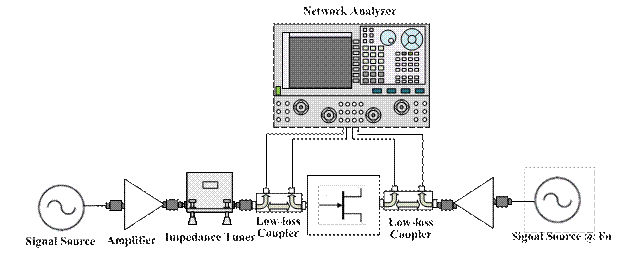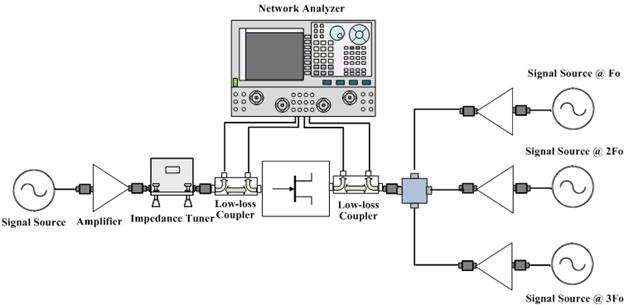Hybrid-active load pull (or hybrid load pull) refers to a combination of active and passive load pull techniques.  In general, this allows the best of both worlds to be achieved while minimizing the disadvantages of each.

A fundamental load tuner can be augmented by an active source, using the mechanical tuner as a prematch and lowering the power requirement of the amplifier while still achieving extremely high VSWRs.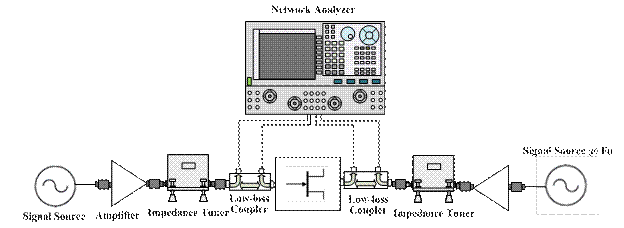A mechanical tuner might be used for fundamental tuning under high powers, while active tuning chains are used at the harmonic frequencies for harmonic load pull.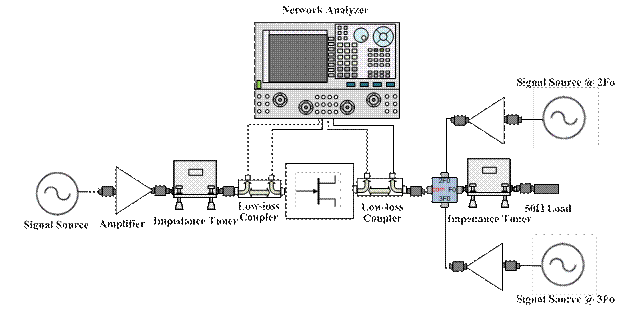Mixed-signal active load pull is a unique type of open-loop active load pull patented by Anteverta Microwave.  Instead of using direct-frequency signal synthesis and analysis, frequency upconverters/downconverters are used in conjunction with extremely wideband ADCs and DACs.  Signals are created at baseband (DC-120 MHz) and then upconverted to RF frequency of operation and injected into the DUT.  The resulting signal from the DUT is then downconverted and analyzed.
Advantages of mixed-signal active load pull include all those listed for open-loop active load pull, and in addition include speed, with the ability to measure up to 1000 impedance/power states per minute, and wideband impedance control.  Because wideband ADCs and DACs are used, modulated signals with bandwidths up to 120 MHz can be created and analyzed, and the impedances over the entire 120 MHz can be individually controlled.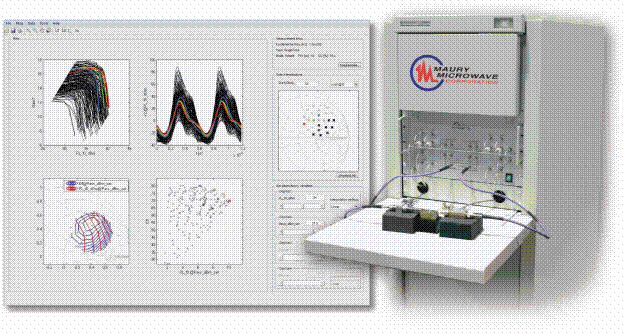A Sample of Load Pull Results
In the first example, a- and b-waves are measured at a predefined set of load impedances (upper right Smith Chart) and useful parameters such as power, gain, efficiency.. are calculated in real-time (upper left table).  Iso-contours of output power are plotted in real-time as the measurement is made (center Smith Chart), and the large-signal input impedance of the DUT is tracked in real-time (upper left Smith Chart).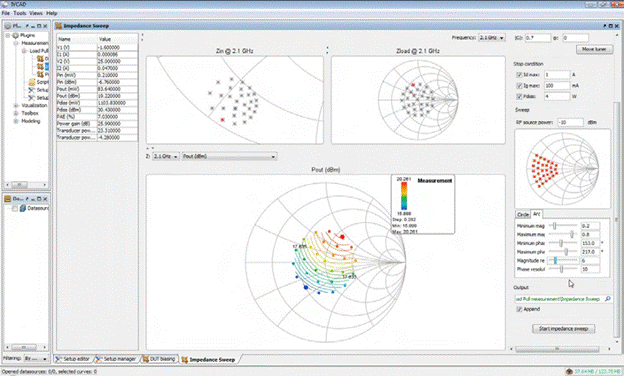In the second example, , a- and b-waves are measured at a predefined set of load impedances (upper right Smith Chart), and for each load impedance the input power is swept over a predefined range.  Useful parameters such as power, gain, efficiency... are calculated in real-time (upper left table).  Power sweep curves at each impedance state are plotted in real-time as the measurement is made (center Smith Chart), and the large-signal input impedance of the DUT is tracked in real-time for each load and power state (upper left Smith Chart).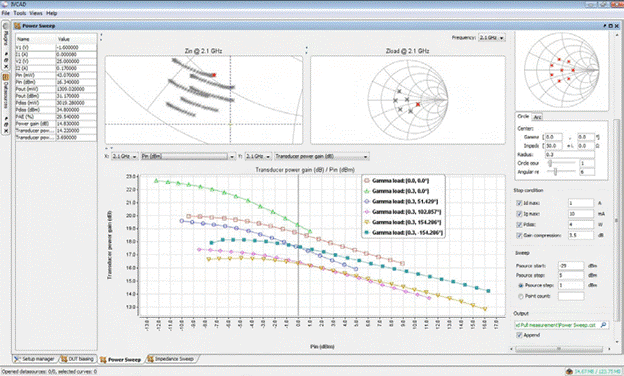Author : Unknown Editor